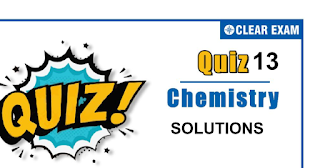## SOLUTIONS Quiz-13

Dear Readers, The one subject in NEET which is candidates who can easily attain good marks is Chemistry. That's the reason, often, one doesn’t pay notice and choose to compromise it. But if one wants to rank above others, the tip is to be thorough with NEET chemistry concepts. The understanding of reactions and definite basic understanding is what requires major attention in Chemistry but once done it only gets simpler from there. The main focus on the to-do list should be on getting a hang of the NCERT syllabus of NEET chemistry.

Q1.  In a solution of 7.8 g benzene (C6H6) and 46.0 g toluene (C6H5CH3), the mole-fraction of benzene is
•   1/2
•   1/3
•   1/5
•   1/6
Solution
Mole fraction of C6H6 = (7.8/78) /(7.8/ 78 + 46 /92) = 1/6

Q2. A thermometer which can be used only for accurate measurement of small differences in temperature is known as a:
•   Beckmann thermometer
•   Contact thermometer
•   Clinical thermometer
•   Platinum resistance thermometer
Solution
Beckmann thermometers do not read actual b. p. or f. p., but they give b. p., f. p. values on their scale.

Q3.   A 0.001 molal solution of [Pt(NH3)4Cl4] in water has a freezing point depression of 0.0054 ͦC. If 𝐾f for water is 1.80, the correct formulation of the above molecule is :
•    [Pt(NH3)4Cl3]Cl
•   [Pt(NH)4Cl2]Cl2
•   [Pt(NH3)4Cl]Cl3
•   [Pt(NH3)4Cl4
Solution
[Pt(NH3)4Cl4] =Gives 𝑛 moles of ions on complete ionization, 𝑖.𝑒.,α = 1 ∆𝑇 = 𝐾𝑓 × molality × (1 − 𝛼 + 𝑛𝛼) 0.0054 = 1.80 × 0.001 × (𝑛)
∴ 𝑛 = 3 Thus, [Pt(NH3)4 Cl2]Cl2 ⟶ [Pt(NH3)4Cl2]2+ + 2Cl- 1 0 0 1 0 2
∴ 𝑛 = 3

Q4.  The molal elevation constant for water is 0.52. What will be the boiling point of 2 molar sucrose solution at 1 atm pressure? (Assume b.p. of pure water is 100℃)
•   101.04℃
•   100.26℃
•   100.52℃
•   99.74℃
Solution
∆𝑇b = 𝑘b × 𝑚𝑜𝑙𝑎𝑙𝑖𝑡𝑦 for dilute solution molarity=molality=2(given) and 𝑘b = 0.52(given)
∴ ∆𝑇𝑏 = 0.52 × 2 = 1.04℃
Now , ∆𝑇b = boiling point of solution – boiling point of solvent ( i.e., 𝐻2𝑂)
∴ boiling point of solution =∆𝑇𝑏 + 𝑏.𝑝𝑡 𝑜𝑓 𝐻2𝑂 =1.04+100
=101.04℃

Q5. At certain temperature a 5.12% solution of cane sugar is isotonic with a 0.9% solution of an unknown solute. The molar mass of solute is
•   60
•   46.17
•   120
•   90
Solution
“Solutions having same osmotic pressure are called isotonic solutions.” The osmotic pressure is given as ∴ 𝜋 = 𝑤𝑏𝑅𝑇 𝑉𝑀𝐵 𝜋 (cane sugar)=𝜋 (unknown solute) 5.12/ 342 = 0.9 /𝑀 M= 342×0.9/5.12 =60 .

Q6.  Molarity of 0.2 N H2SO4 is
•   0.1
•   0.2
• 0.3
•   0.4
Solution
Normality of acid=molarity ×basicity 0.2 = 𝑀 × 2 ∴ 𝑀 = 0.2/2 = 0.1

Q7. 3.0 molal NaOH solution has a density of 1.110 g/mL. The molarity of the solution is

•   3.9732
•   2.9732
•   1.9732
•   0.9732
Solution

Molarity(𝑚) = 𝑀 /(1000𝑑 − 𝑀𝑀′)× 1000
Where 𝑀′ =molar mass of solute
3 = 𝑀 × 1000/(1000 × 1.11 − 𝑀 × 40)
1000𝑀 = 3330 − 120 M
1120 𝑀 = 3330
𝑀 = 3330 /1120
= 2.9732

Q8. How many gram of NaOH will be required to prepare 500 g solution containing 10%𝑤/𝑤 NaOH solution
•   100 g
• 50g
•   0.5g
•   5.0
Solution
Given, in 100 g of solution NaOH present = 10 g
∴ In 500 g of solution NaOH present = (10 × 500) /100 =50 g
So, 50 go NaOH will be required to prepare 500 g 10% 𝑤/𝑤 NaOH solution.

Q9. The molal elevation/depression constant depends upon

•   Nature of solvent
•   Nature of solute
•   Temperature
•   ∆𝐻 solution
Solution
It is a characteristic of given solvent

Q10.  The vapour pressure of benzene at a certain temperature is 640 mm of Hg. A non-volatile and nonelectrolyte solid weighing 2.175 g is added to 39.08 g of benzene. If the vapour pressure of the solution is 600 mm of Hg, what is the molecular weight of solid substance?

•   49.50
•   59.60
•   69.60
•   79.82
Solution
Given, vapour pressure of benzene,
𝑝o=640 mm Hg
Vapour pressure of solution,
p =600 mm
Hg Weight of solute, w = 2.175 g
Weight of benzene, W = 39.08 g
Molecular weight of benzene, M=78 g
Molecular weight of solute, m =?
According to Raoult’s law,
𝑃o−𝑃 / 𝑃o = 𝑤×𝑀 / 𝑚×𝑊
640−600 / 640 = 2.175×78 / 𝑚×39.08
40 / 640 = 2.175×78 /𝑚×39.08
m = 16×2.175×78 / 39.08
m =69.60## Want to know more

Please fill in the details below:

## Latest NEET Articles\$type=three\$c=3\$author=hide\$comment=hide\$rm=hide\$date=hide\$snippet=hide

Name

ltr
item
BEST NEET COACHING CENTER | BEST IIT JEE COACHING INSTITUTE | BEST NEET, IIT JEE COACHING INSTITUTE: SOLUTIONS QUIZ 13
SOLUTIONS QUIZ 13
https://1.bp.blogspot.com/-asqfQgC7UWQ/X70UwJrY8nI/AAAAAAAAEZ4/r67PUUEoWbghcs_CbKf4GfuC_JVqYunIgCLcBGAsYHQ/s320/hydrocarbons%2B1%2B%25282%2529.png
https://1.bp.blogspot.com/-asqfQgC7UWQ/X70UwJrY8nI/AAAAAAAAEZ4/r67PUUEoWbghcs_CbKf4GfuC_JVqYunIgCLcBGAsYHQ/s72-c/hydrocarbons%2B1%2B%25282%2529.png
BEST NEET COACHING CENTER | BEST IIT JEE COACHING INSTITUTE | BEST NEET, IIT JEE COACHING INSTITUTE
https://www.cleariitmedical.com/2020/11/solutions-quiz-13.html
https://www.cleariitmedical.com/
https://www.cleariitmedical.com/
https://www.cleariitmedical.com/2020/11/solutions-quiz-13.html
true
7783647550433378923
UTF-8